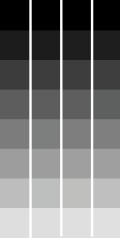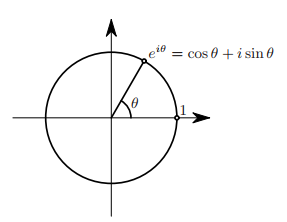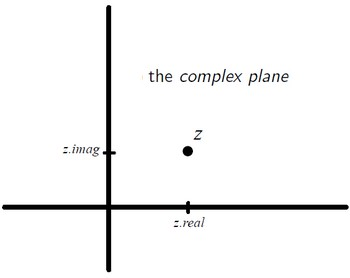# Number System - Complex Number -

A real number plus an imaginary number is a complex number.

Complex numbers are a number system.

A complex number has a real part and an imaginary part. $$\text{complex number} = \text{(real part)} + \text{(imaginary part)} i$$

where:

Complex Numbers are the intellectual ancestors of vectors.

Complex numbers are convenient to apply geometric transformation (such as rotation, scaling and translation) in two dimensions.

## Visualization

Since any complex number is specified by two real numbers one can visualize them by plotting a point with coordinates (a,b) in the plane for a complex number a + bi. The plane in which one plot these complex numbers is called the Complex plane, or Argand plane.

• Real numbers lie on the horizontal axis
• Imaginary numbers lie on the vertical axis

## Properties

• Length: |z| is the distance from the origin to the point z in the complex plane.

$$|z| = \sqrt {a^2 + b^2}$$

• Angle: The angle θ is called the argument of the complex number z. Notation:

$$arg z = θ$$

## Law

when you multiply complex numbers:

• their lengths get multiplied
• and their arguments get added.

## Example

• Problem: $(x -1)^2 = -9$
• Solution: $x = 1 + 3 i$

## Documentation / Reference

Discover MoreLinear Algebra - Vector

tuple in Linear algebra are called vector. A vector is a list of scalar (real number) used to represent a When the letters are in bold in a formula, it signifies that they're vectors, To represent...Mathematics - Complex Exponential (Euler's formula)

Euler's formula provides a powerful connection between analysis and trigonometry, and provides an interpretation of the sine and cosine functions (the sinusoidal functions) as weighted sums of the exponential...Number - Field

A field is a collection of “numbers” with the operator: +, -, , / Different fields are like different classes obeying to the same interface. Same as ?? A field is also known as abody: corps...
Number System (Classification|Type)

Numbers are classified according to how they are represented or according to the properties that they have. They have what's called a type. For systems for expressing numbers, see Numeral system. ...
Number System - Imaginary Number

An imaginary numberi is one solution to and is a part of the definition of a complex number It was invented because formulas sometimes required the manipulation of square roots of negative numbers....Number, Numeric, Quantity

A number is a mathematical object used to: count, label, and measure. Example: When we are interested in the total number of each species of tortoise, or how many individuals there are per square...Python - Complex Numbers

complex numbers in Python Python uses 'j' for the imaginary unit, not 'i'. Type Complex Addition The real and imaginary parts of a complex number can be interpreted as x and y coordinates...R - Complex Number

A vector containing complex number (for example: 1+2i)